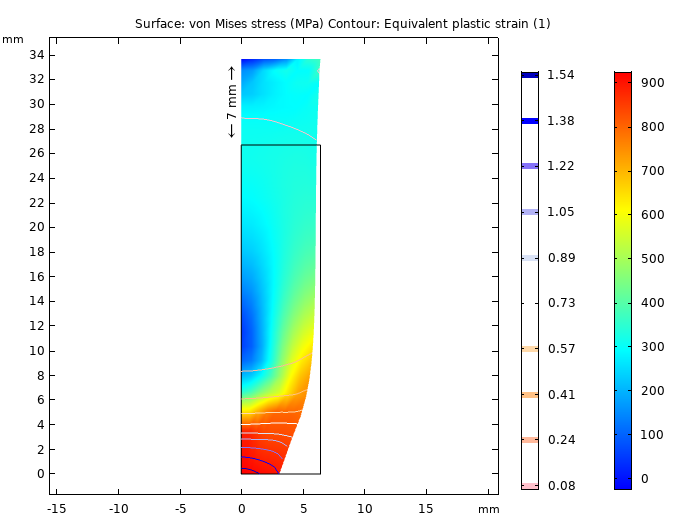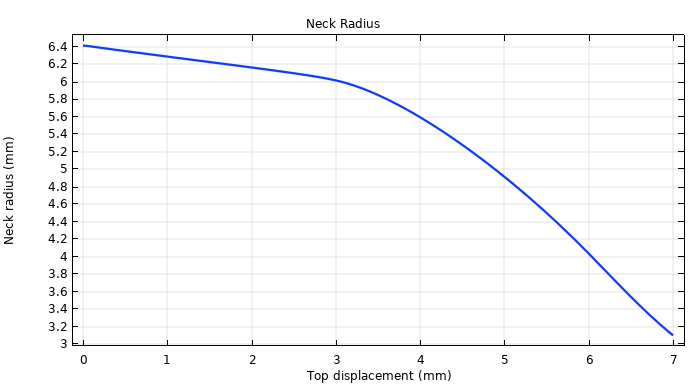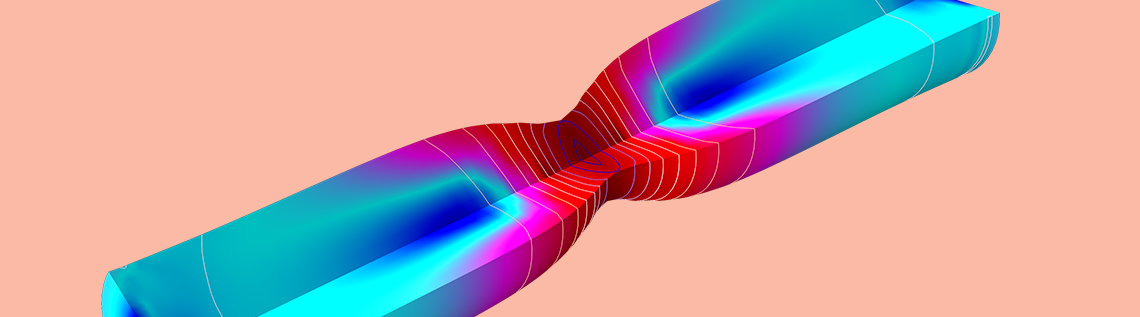# 弹塑性金属条颈缩基准模型评估

2019年 3月 18日

### 对弹塑性材料进行单轴试验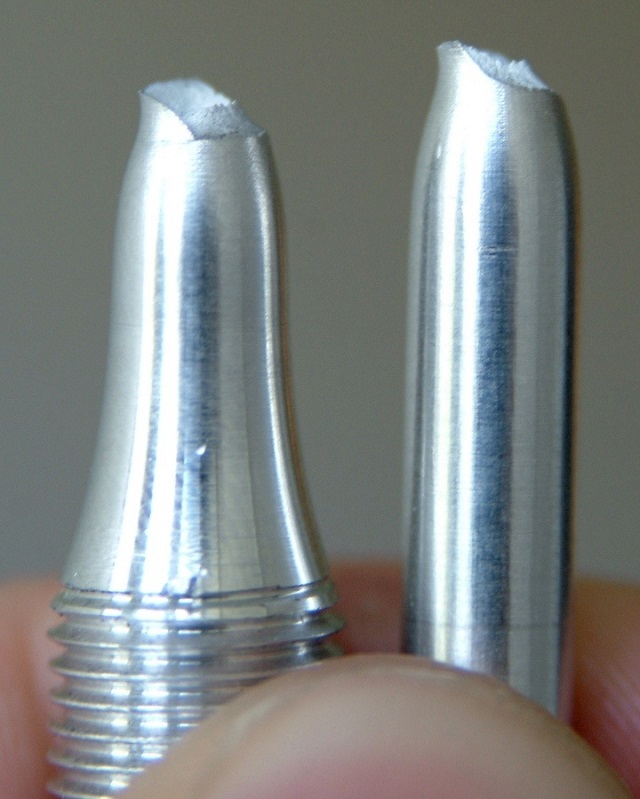### 浅谈颈缩不稳定性背后的理论

L \cdot A = L_0 \cdot A_0 = V_0

A dL + L dA = 0

d \epsilon = \frac{dL}{L}

\sigma = \frac{F}{A}

0 = dF = d(A \sigma) = A d\sigma + \sigma dA

\frac{d\sigma}{\sigma}=-\frac{dA}{A}=\frac{dL}{L}=d\epsilon

\frac{d\sigma}{d\epsilon}= \sigma

### 用 COMSOL® 软件模拟弹塑性金属条的颈缩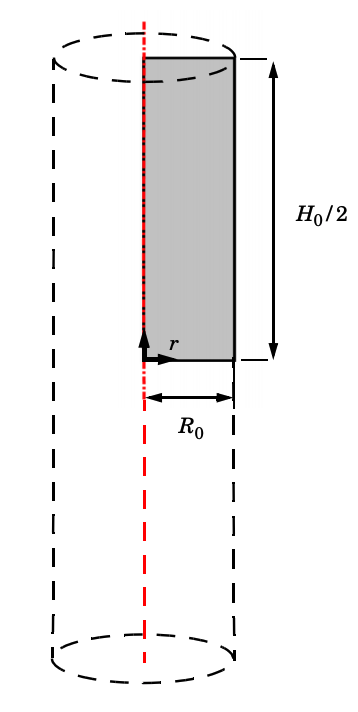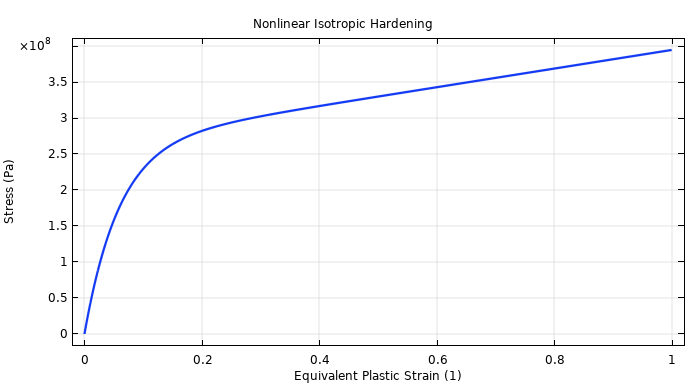### 评估仿真结果